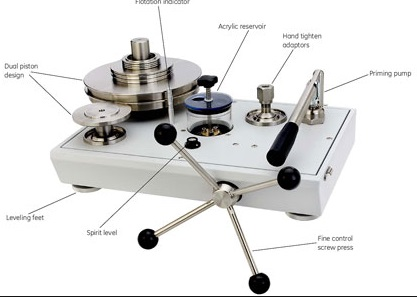# Working of Dead Weight Tester## Description

The dead weight tester apparatus consists of a chamber that is filled with oil-free impurities and a piston-cylinder combination is installed on top of the chamber as shown in the diagram. The upper part of the piston is connected to a platform for loading weights. A piston with handle has been provided to vary the pressure of the oil in the chamber. The manometer to be tested is installed on an appropriate plate.

## Operation

The dead weight tester is basically a device for measuring pressure and producing pressure. It is used to calibrate manometers. The following procedure is adopted to calibrate manometers. Gauge calibration means entering a precisely known pressure sample into the manometer under test and then observing the response of the manometer. To create this known pressure accurately, the following steps are followed.

The valve of the apparatus is closed.A known weight is placed on the platform.

Now by operating the plunger, fluid pressure is applied to the other side of the piston until enough force is developed to lift the piston-weight combination. When this happens, the piston weight combination floats freely within the cylinder between limit stops.

In this condition of equilibrium, the pressure force of fluid is balanced against the gravitational force of the weights plus the friction drag.

Therefore, PA = Mg + F

Hence : P = Mg + F / A

where, P = pressure

M = Mass; Kg

g = Acceleration due to gravity ; m/s²

F = Friction drag; N

A = Equivalent area of piston – cylinder combination; m²

Thus the pressure P which is caused due to the weights placed on the platform is calculated.

After calculating P , the plunger is released.

Now the pressure gauge to be calibrated is installed in an appropriate place in the dead weight tester. The same known weight that was used to calculate P is placed on the platform. Due to the weight, the piston moves downwards and exerts a pressure P on the fluid. Now the valve in the device opens so that the fluid pressure P is transmitted to the meter, which causes the meter to indicate a pressure value. This pressure value shown on the indicator must be equal to the known inlet pressure P. If the indicator indicates any other value than p, the indicator is set to read a value equal to p. Therefore, the gauge is calibrated.

### Applications:

It is used to calibrated all kinds of pressure gauges such as industrial pressure gauges, engine indicators and piezoelectric transducers.

it is simple in construction and easy to use.

It can be used to calibrated a wide range of pressure measuring devices.

Fluid pressure can be easily varied by adding weights or by changing the piston cylinder combination.

### Limitations:

the accuracy of the dead weight tester is affected due to the friction between the piston and cylinder, and due to the uncertainty of the value of gravitational constant ‘g’.